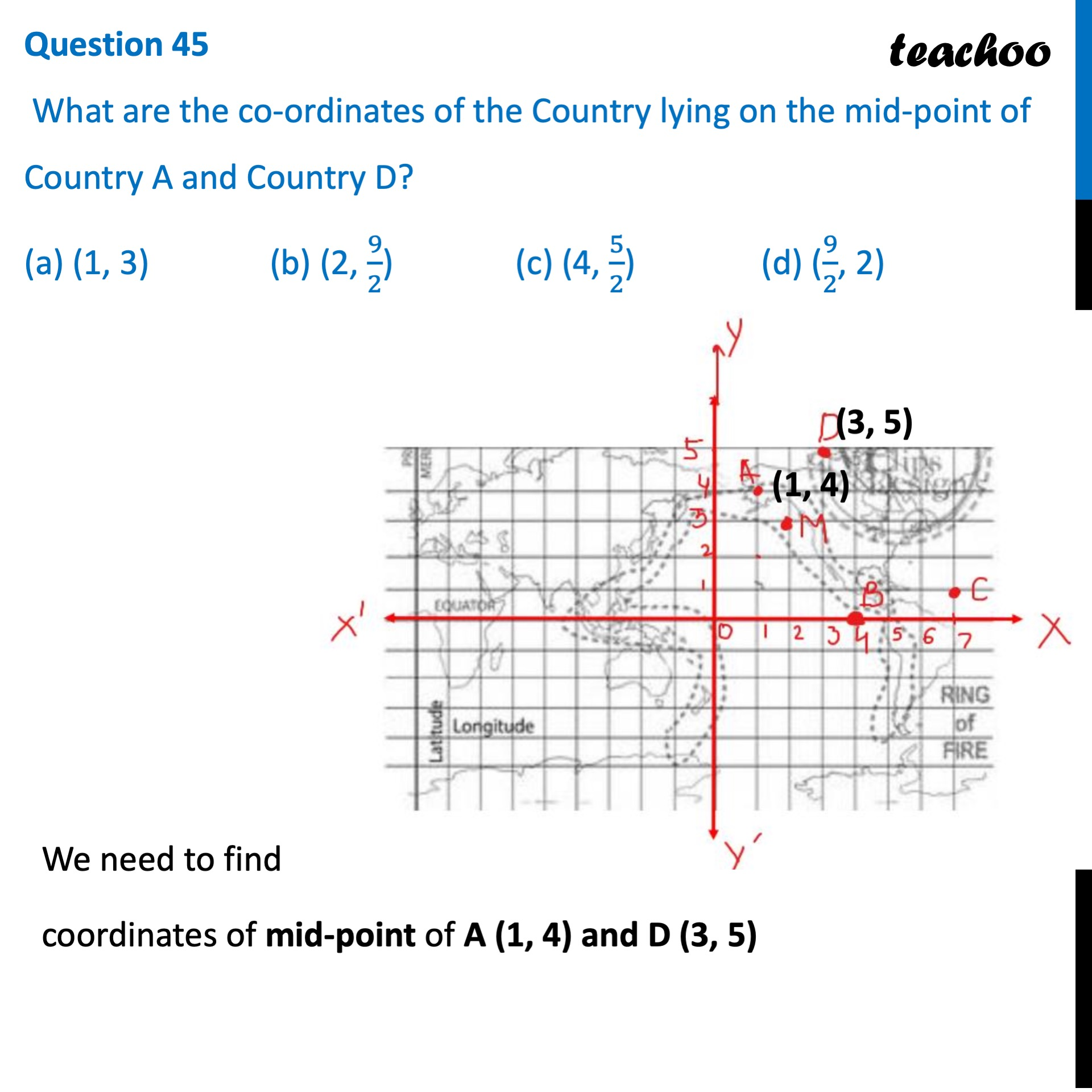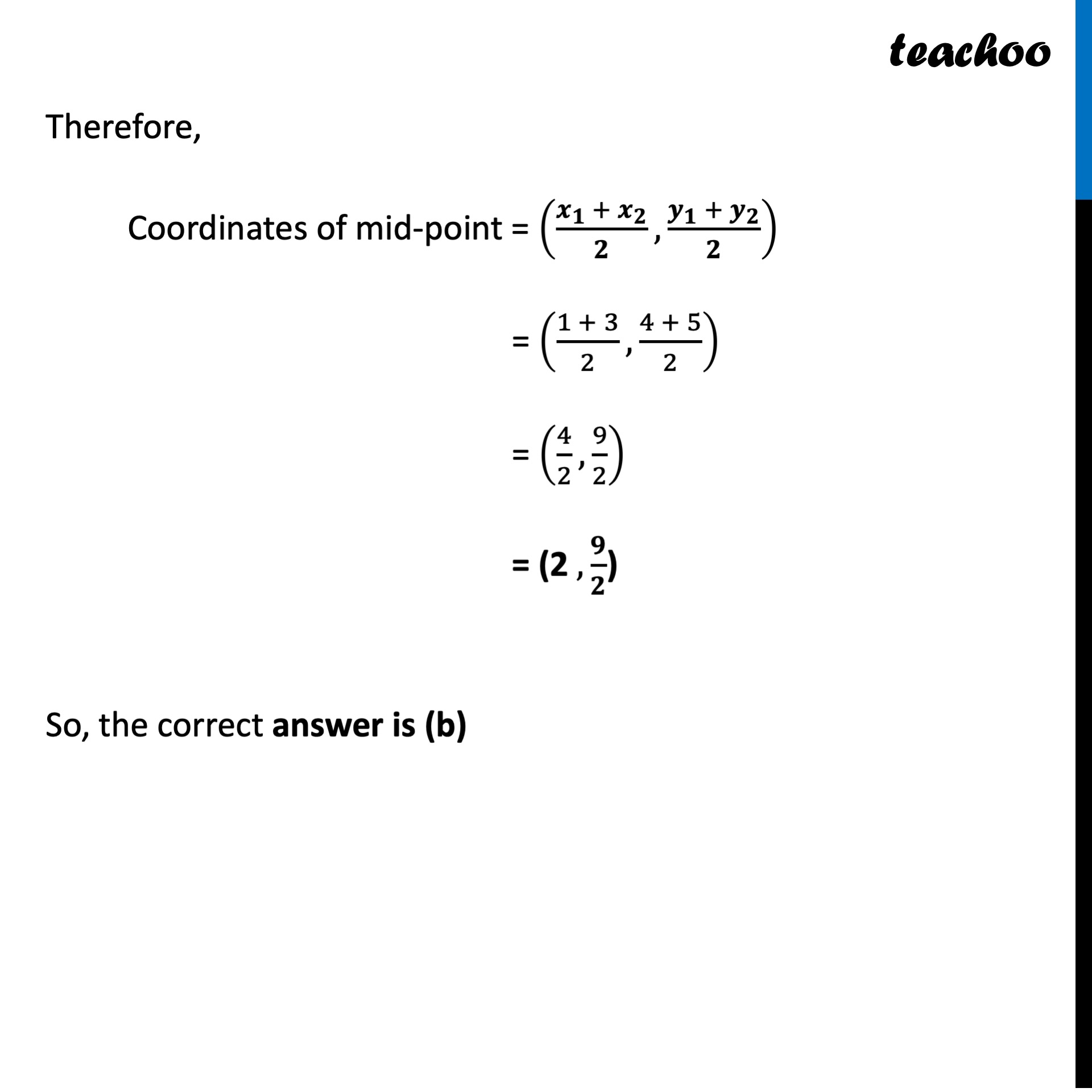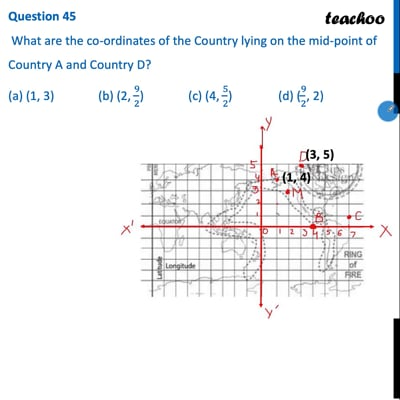CBSE Class 10 Sample Paper for 2022 Boards - Maths Basic [MCQ]

Class 10
Solutions of Sample Papers for Class 10 Boards

## (a) (1, 3)   (b) (2, 9/2)   (c) (4, 5/2)     (d) (9/2, 2)This video is only available for Teachoo black users

Introducing your new favourite teacher - Teachoo Black, at only ₹83 per month

### Transcript

Question 45 What are the co-ordinates of the Country lying on the mid-point of Country A and Country D? (a) (1, 3) (b) (2, 9/2) (c) (4, 5/2) (d) (9/2, 2) We need to find coordinates of mid-point of A (1, 4) and D (3, 5) Therefore, Coordinates of mid-point = ((𝒙_𝟏 + 𝒙_𝟐)/𝟐,(𝒚_𝟏 + 𝒚_𝟐)/𝟐) = ((1 + 3)/2,(4 + 5)/2) = (4/2,9/2) = (2 ,𝟗/𝟐) So, the correct answer is (b)